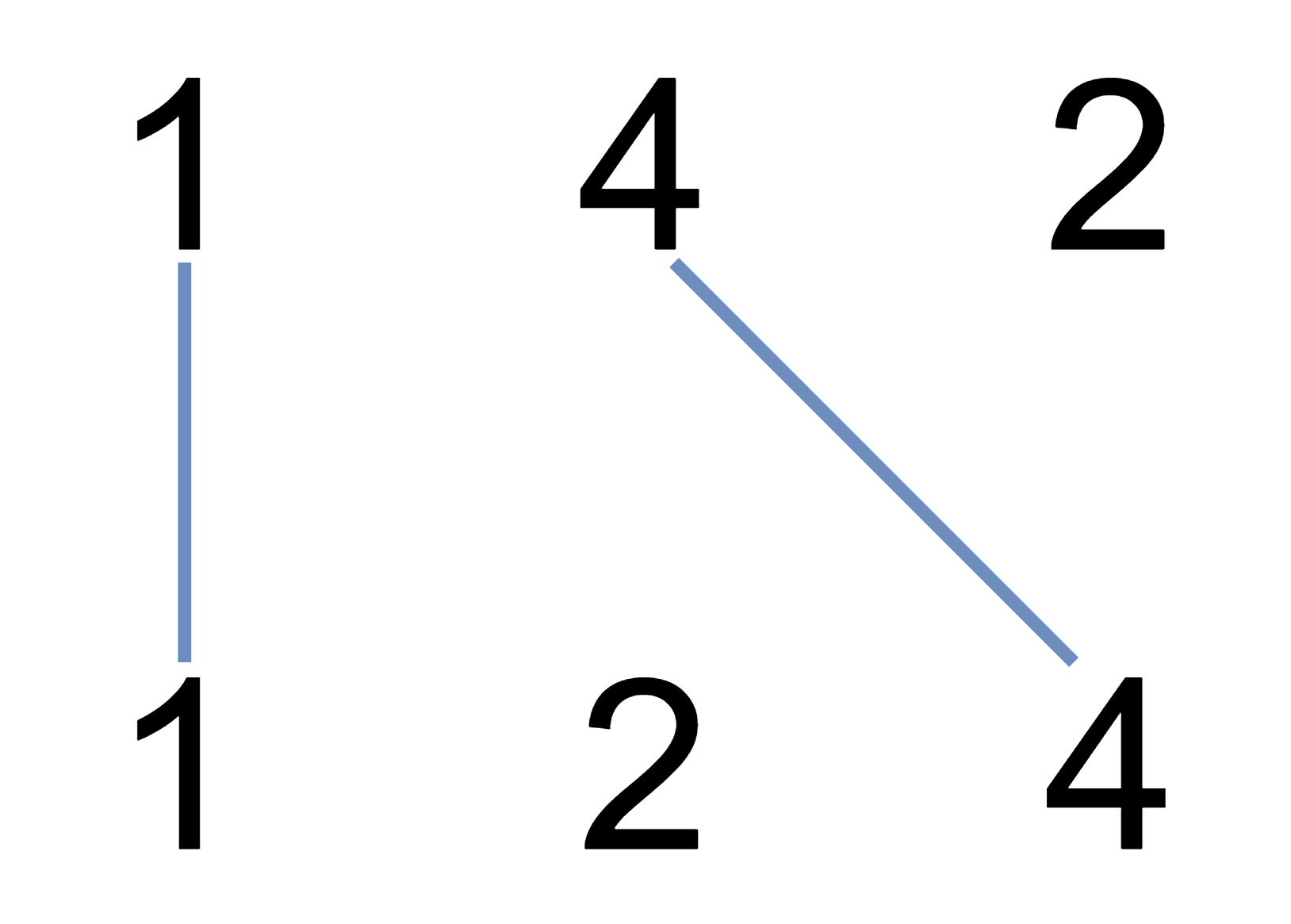Amazon

2020-05-25

## 1035. Uncrossed Lines

### Question:

We write the integers of `A` and `B` (in the order they are given) on two separate horizontal lines.

Now, we may draw connecting lines: a straight line connecting two numbers `A[i]` and `B[j]` such that:

• `A[i] == B[j]`;
• The line we draw does not intersect any other connecting (non-horizontal) line.

Note that a connecting lines cannot intersect even at the endpoints: each number can only belong to one connecting line.

Return the maximum number of connecting lines we can draw in this way.

#### Example 1:``````Input: A = [1,4,2], B = [1,2,4]
Output: 2
Explanation: We can draw 2 uncrossed lines as in the diagram.
We cannot draw 3 uncrossed lines, because the line from A=4 to B=4 will intersect the line from A=2 to B=2.
``````

#### Example 2:

``````Input: A = [2,5,1,2,5], B = [10,5,2,1,5,2]
Output: 3
``````

#### Example 3:

``````Input: A = [1,3,7,1,7,5], B = [1,9,2,5,1]
Output: 2
``````

Note:

1. `1 <= A.length <= 500`
2. `1 <= B.length <= 500`
3. `1 <= A[i], B[i] <= 2000`

### Solution:

Using the exactly same DP idea as LeetCode Question 1143 to find the longest common subsequence which can avoid crossing.

``````class Solution {
public int maxUncrossedLines(int[] A, int[] B) {
int n = A.length;
int m = B.length;
if (m > n) return maxUncrossedLines(B, A);
int[][] dp = new int[m+1];
for (int i = 1; i <= n; i++) {
int index = i % 2;
for (int j = 1; j <= m; j++) {
if (A[i - 1] == B[j - 1]) {
dp[index][j] = dp[(i - 1) % 2][j - 1] + 1;
} else {
dp[index][j] = Math.max(dp[(i - 1) % 2][j], dp[index][j - 1]);
}
}
}
return dp[n % 2][m];
}
}
``````